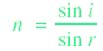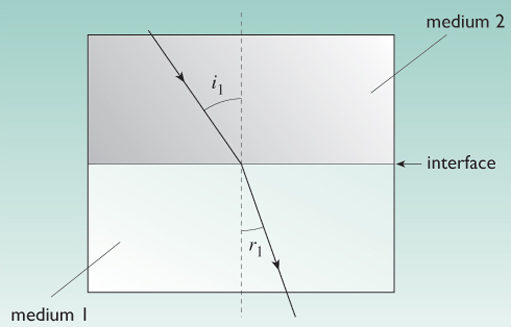Science, Maths & Technology

### Become an OU studentManufacturing

Start this free course now. Just create an account and sign in. Enrol and complete the course for a free statement of participation or digital badge if available.

# 7.8 Optical terms

## 7.8.1 Refractive index

Refractive index (given the symbol n) is an important optical property, as it is a measure of how much a particular material can change the direction of a ray of light (i.e. refract it) as it passes through that material. A high refractive index material will change the direction much more than a low one, so the material for a spectacle lens, which has to bend the light rays to the correct angle for the eye, can be made thinner.

Refractive Index, n, can be expressed as the change in the angle of light passing into a material, hencewhere i is the angle that the light makes when it is incident ('shining') on the material and r is the angle that it is refracted through (Figure 57).Figure 57 Change in direction of light passing from one material to another